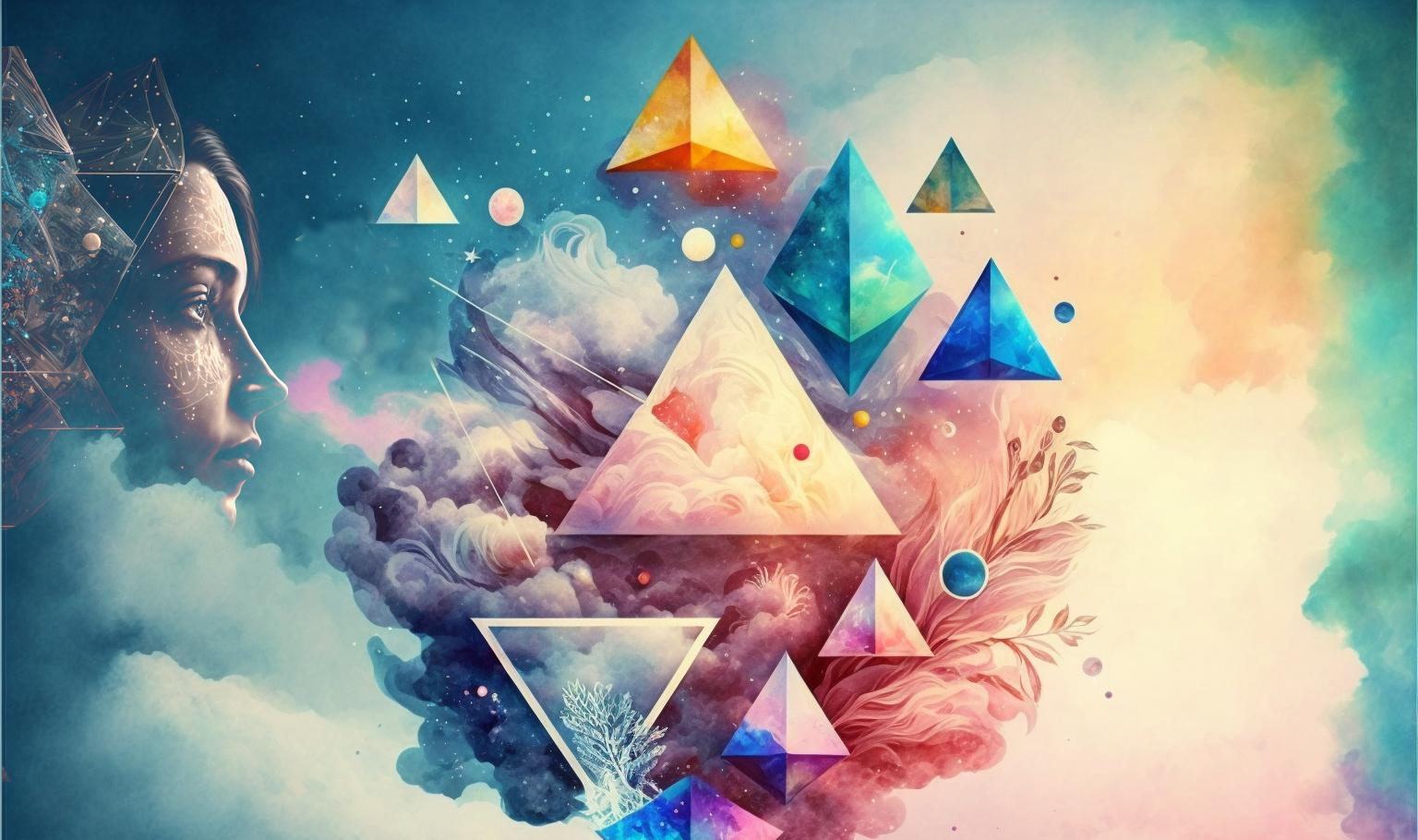The First Personality Reading Based On
The Sacred Geometry Of Your Name Symbols.

# Esoteric teaching of Pythagoras

The number, the symbol and the idea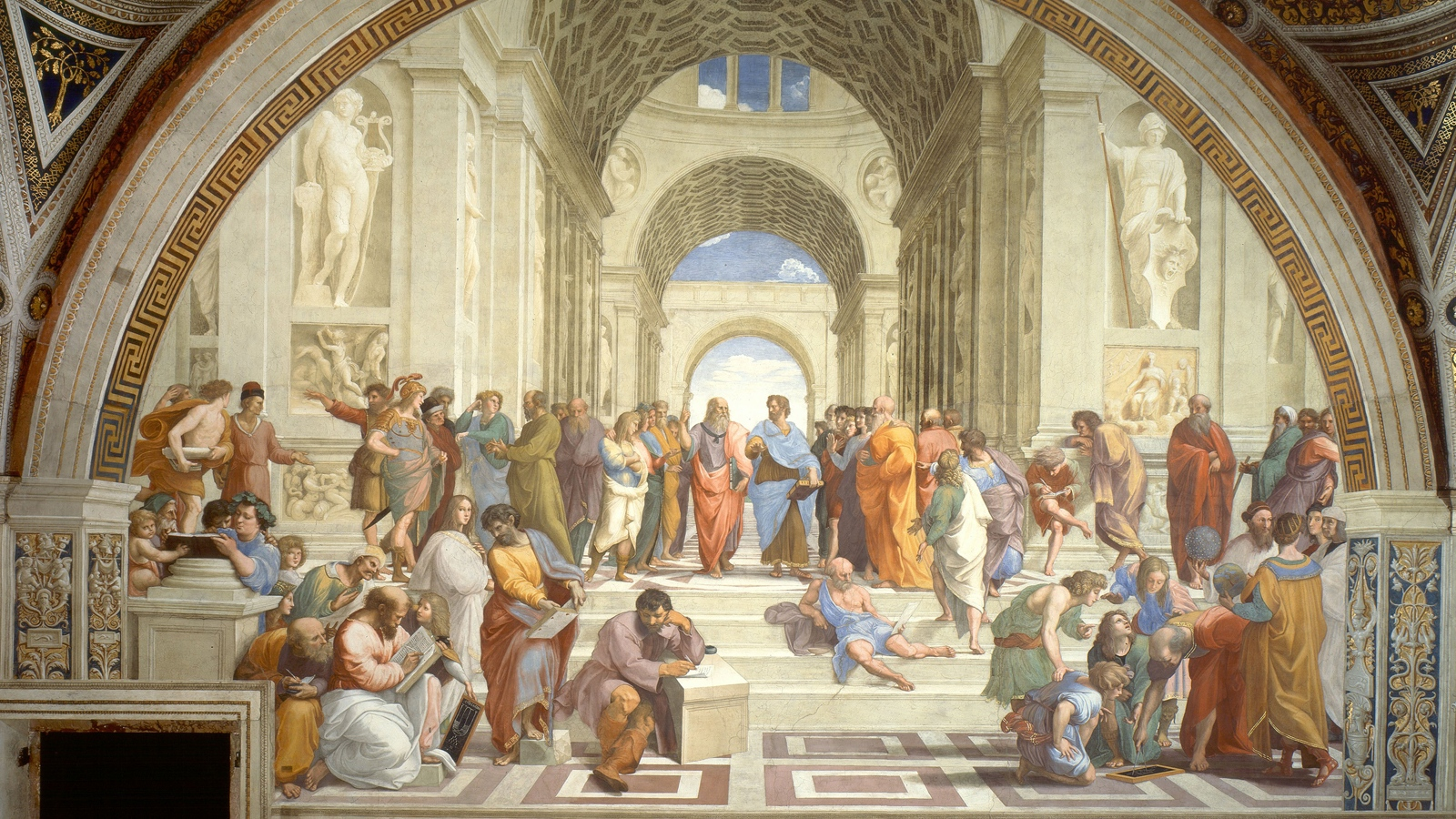## REDUCED NUMBERS

All numbers can be reduced to
the first 9 single-digit numbers.
Ex : 127 = 1 + 2 + 7 = 10
et 10 = 1 + 0 = 1
The number 127’s reduction

is the number 1.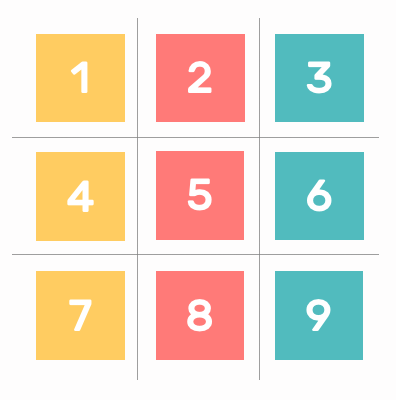FIND OUT MORE

## FIGURATE NUMBERS

Numbers can be represented
in space as a function of
the number of units they contains.
Ex : number 3 contains 3 units.
It can be drawn from three points
or a triangle.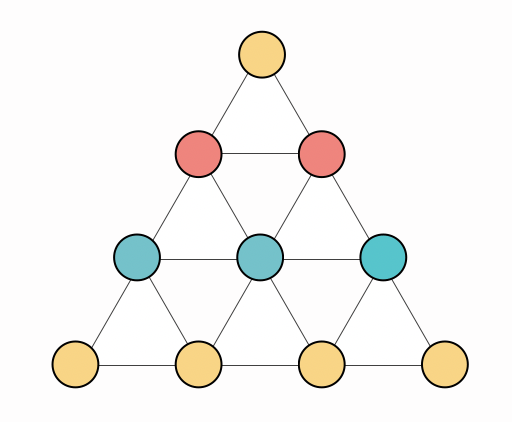FIND OUT MORE

## IDEA-NUMBERS

Pythagoras, who coined the term "cosmos", defined the Three Worlds as:
• World of Archetypes (which later became the Theory of Ideas with Plato);
• Microcosm, or small world, Man;
• Macrocosm, the large world, the Universe.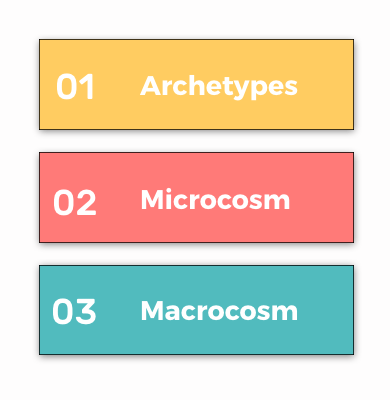FIND OUT MORE

### The ancient numerology of Pythagoras

The number, the symbole and the idea

Geonumerology is an interdisciplinary approach which aims to restore the ancient numerology of Pythagoras to its former glory. It is therefore at the heart of the teaching of this philosopher's teaching that Geonumerology finds its source.

Arithmetic and geometry were, along with astrology and music, the four sciences that were taught at the time within the framework of the Quadrivium.

Pythagoras is certainly the first great philosopher of the Western tradition. It was he who invented the word philosophy. Everyone knows Pythagoras for his multiplication tables or his theorem. But what is less known is that his mathematics actually leads to a metaphysics, being convinced that numbers are the principle and the key to the entire universe.

His main idea is to have considered the universe as an ordered whole, a "Cosmos", where the numbers serve as a support for a vibratory reality. We can therefore legitimately consider Pythagoras as the father of current physics.

As Man, or microcosm, is the image of the universe, the macrocosm, the laws that apply to one also apply to the other.

The initiatory process which characterizes his teaching is related to the realisation of the Self in psychology as well as to the quest for the philosopher's stone of the alchemists. These three paths invite us to direct our gaze inward, in a process of introspection to the discovery of our soul.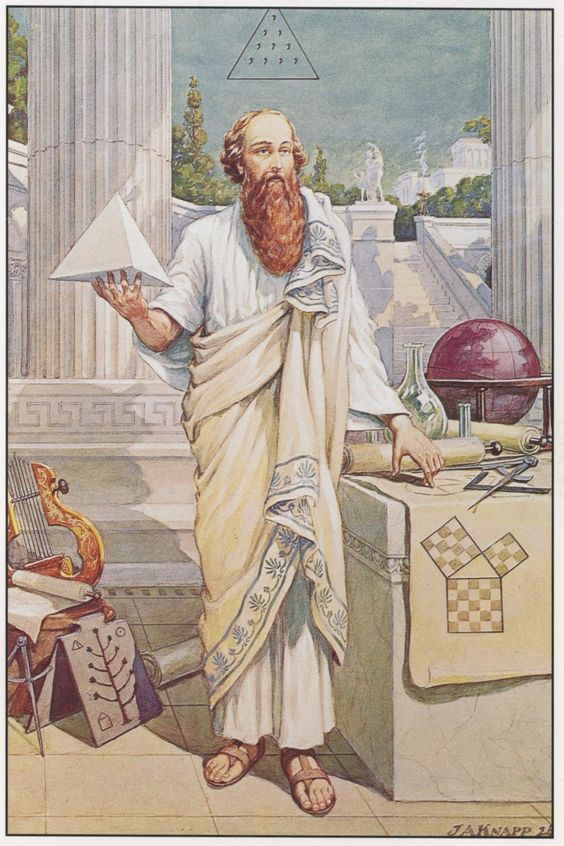## The 9 numbers numerology

The reduced number10 = 1 + 0 = 1
11 = 1 + 1 = 2
12 = 1 + 2 = 3
13 = 1 + 3 = 4
14 = 1 + 4 = 5
15 = 1 + 5 = 6
16 = 1 + 6 = 7
17 = 1 + 7 = 8
18 = 1 + 8 = 9
...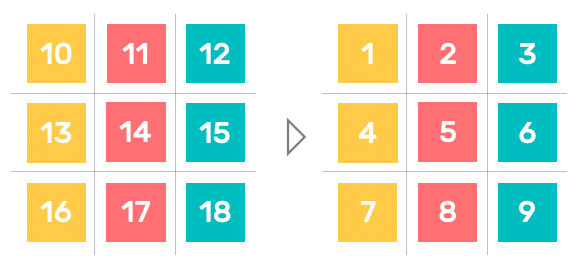FIND OUT MORE

### The principle of reduction

The theosophical reduction (from the Greek: theos, divine or Gods and sophia, wisdom) corresponds to the current 9-numbers of numerology. It consists in reducing all numbers to the first 9.

### The principle of correspondence

« The theosophical reduction was an operation familiar to Pythagoreans who neglected numbers greater than 10, so they reduced numbers greater than 10 to the first nine numbers, taking into account only their root or pythmen, i.e., substituting the remainder of their division by nine, or the number nine even when the number was a multiple of nine. »

Arthuro Reghini ~ Sacred Numbers in the Pythagorean Masonic Tradition

In the time of Pythagoras (6th century B.C.), the letters of the Greek alphabet were used to designate numbers: Alpha for One, Beta for Two, Gamma for Three, Delta for 4, and so on.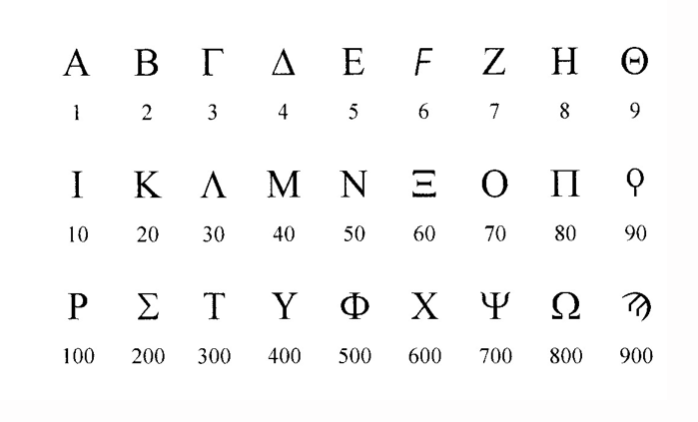Numerology derives from this principle and applies a numerical value to each letter of your name which making it possible to calculate the number for any name or word by adding together the values of its letters.

### The Matrix Layout

This is the layout adopted in geonumerology and derived directly from the tables created by Pythagoras.

The interest of such an arrangement is to allow a cartesian approach (abscissa and ordinate) of the first 9 numbers, where each one has a place assigned to it in relation to the whole.

### Representative numbers

The numbers 1, 5 and 9 keep their place in the matrix whatever the arrangement, in rows or columns. They are representative numbers.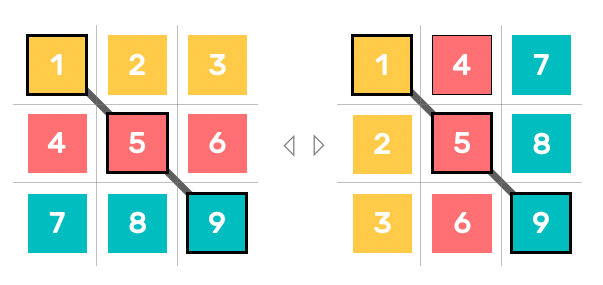## Figurate numbers

From number to shapesThe Tetractys from Pythagoras

### Pythagorean geometry

Figurate numbers allow us to create a bridge between arithmetic and geometry. They derive from the use of small pebbles represent the units that make up numbers with symmetrical geometric shapes.

Pythagoras was the first to make the connection between numbers, geometric shapes and spatial dimensions. Below is a visualisation of the number 10, the fourth triangular number and central symbol of the Pythagorean school, named the Tetractys.

« They saw numbers as a stream, a flow of units or monads, reflecting the infinite multiplication of one and the same divine Unit. These units, represented by points or groups of points, were translated in the forms of the sensible world by geometrical figures, in two or three dimensions. »

Matila Ghyka ~ The Golden Number
FIND OUT MORE

### Tetrahedron, symbol of number 4

Given the total parallelism between arithmetic and geometry, it is thus possible to visualize the number in space using points, or pebbles (calculi in Latin), according to the number of units it contains:

• 1 point for the number 1;
• 2 points for the number 2, the line (1D);
• 3 points for the number 3, the triangle (2D);
• 4 points for the number 4, the tetrahedron (3D).

A figured number is an integer that is usually represented by points and which forms a regular geometric figure.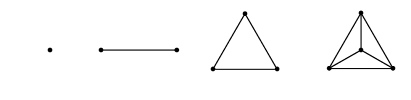Geonumerology is therefore a mixture of numerology and geometry. Geometry will allow us to figure the number, to give it body in space, to grasp its lines of force and finally to discover the idea hidden behind the symbol.

### Theosophical addition & triangular numbers

To know the Theosophical addition of a number, it is enough to add up arithmetically all the numbers from the unit to it.
Thus the number 4 gives in theosophical addition: 1 + 2 + 3 + 4 = 10.

This operation corresponds in all respects to the way Triangular numbers (1, 3, 6, 10, ...) are elaborated.

1 = 1
2 = 1 + 2 = 3
3 = 1 + 2 + 3 = 6
4 = 1 + 2 + 3 + 4 = 10
5 = 1 + 2 + 3 + 4 + 5 = 15
6 = 1 + 2 + 3 + 4 + 5 + 6 = 21
7 = 1 + 2 + 3 + 4 + 5 + 6 + 7 = 28
8 = 1 + 2 + 3 + 4 + 5 + 6 + 7 + 8 = 36
9 = 1 + 2 + 3 + 4 + 5 + 6 + 7 + 8 + 9 = 45
The first 9 triangular numbers

The Number represents the Idea. He is the Father. Geometry is the Mother of Creation, it generates the forms. Each Number inhabits the form that is "destined" for it. Thus the metaphysical abstraction of the Number materializes in geometry, it takes shape.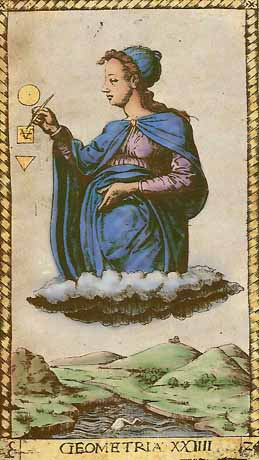« Each number responds to a characteristic idea and hieroglyph, so that the laws of number combinations verify the combination of symbols and ideas. (...) This law is one of the best keys to open the ancient mysteries. »

Édouard Schuré ~ The Great Initiates

## The Three Worlds

Archetypes, Microcosm and Macrocosm1 = 1
3 = 3
6 = 6
10 = 1 + 0 = 1
15 = 1 + 5 = 6
21 = 2 + 1 = 3
28 = 2 + 8 = 1
36 = 3 + 6 = 9
45 = 4 + 5 = 9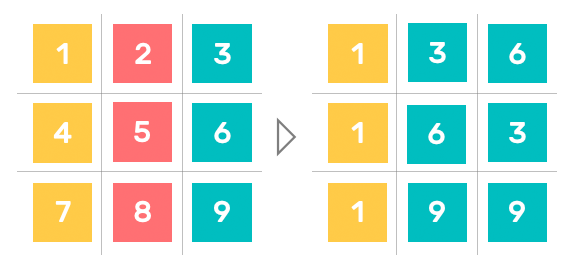FIND OUT MORE

### Secret value of numbers

All integers are associated with one of the following 4 secret values: 1, 3, 6 or 9.

The secret value of a number is obtained by applying the process of theosophical addition to it followed by reduction.

Let's take an example: the number 7

• The theosophical addition of the number 7 gives: 1 + 2 + 3 + 4 + 5 + 6 + 7 = 28
• The theosophical reduction of the number 28 is : 2 + 8 = 10 = 1 + 0 = 1
• The secret value of 7 is therefore at 1

### Worlds and reflections

If the numbers 4 and 7 are the refections of the Divine World in the Human World and in the Natural World, then there must be a human reflection in the Divine and Natural Worlds as well as a natural reflection in God and Man .

### The law of analogy

The keystone of the esoteric teaching of Pythagoras rests on the idea of the interdependence of all the elements of the cosmos by a link, a primordial energy, associated by some with divine love, by others with the law of attraction, in a word with the force that unites, or better, keeps together all parts of the universe.

### The law of one

By applying the secret value to the first nine numbers, we see that the total of each line (see on the right), or World, gives the Unit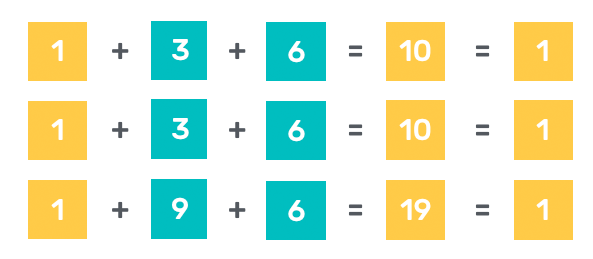The unit reappears every three numbers in the Three Worlds according to a ternary law

The unit and its reflexions in the two other Worlds constitute the Divine Axis of Divine Attributes (first column of the secret matrix composed only of 1) and correspond in the archetypal matrix to the numbers 1, 4 and 7.

« These three progressions represent
the Three Worlds
in which everything is enclosed. »

Pythagoras

## Field of investigation

Topics covered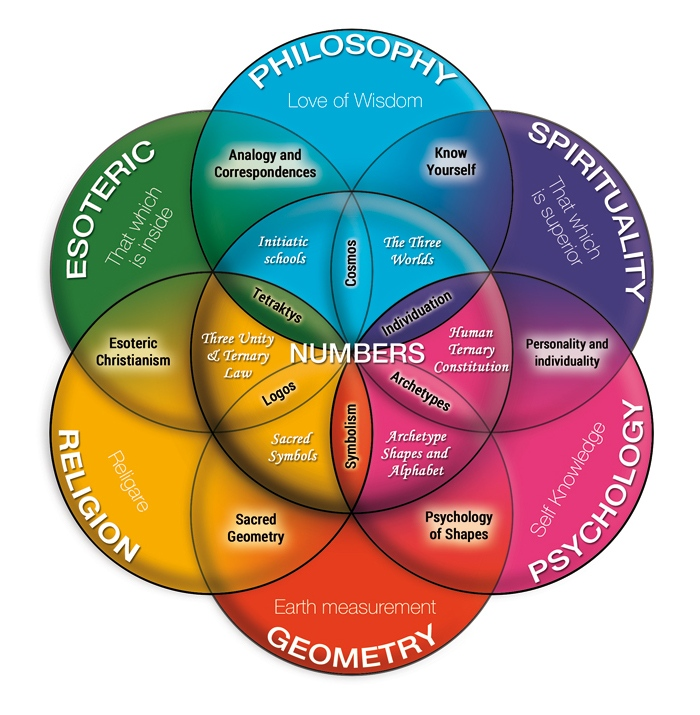Numbers are at the heart of the esoteric teaching of Pythagoras. They represent the archetypal principles on which all things are based.

The qualitative or esoteric study of numbers was the basis of the philosophical teaching of Pythagoras.

The knowledge of oneself led to the knowledge of the universe and the Gods.

The sacred symbols used to transmit the teaching acted on the deep psychology of the individual.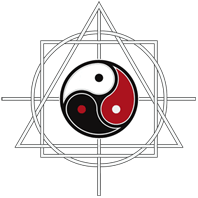THE SACRED GEOMETRY OF YOUR NAME